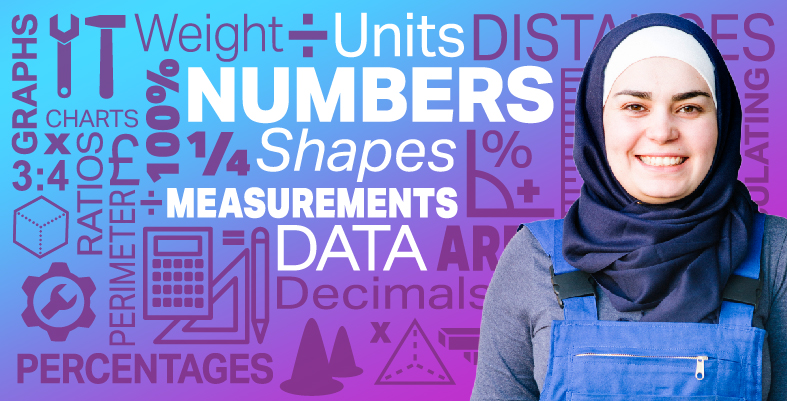Everyday maths for Construction and Engineering 1

Start this free course now. Just create an account and sign in. Enrol and complete the course for a free statement of participation or digital badge if available.

Free course

# 3.1 Weighing things

It’s useful to have an idea of how much things weigh. It can help you to work out the weight of materials or equipment to make sure that you carry out the job safely; for example, if you do not calculate how heavy the roof is on a house, the walls and beams may not support it correctly.

Try estimating the weight of something before you weigh it. It will help you to get used to measures of weight.

Hint: Remember to use appropriate units. Give the weight of small things in grams and of heavy things in kilograms.

Take a look at the example below before having a go at the activity.

## Example: Weighing a screw

1. Which metric unit would you use to weigh an average screw?
2. Estimate how much a screw weighs and then weigh one.
3. How much would 20 of these screws weigh? Would you use the same units?

### Method

1. A screw is quite small, so it should be weighed in grams.
2. How much did you estimate that a screw weighs? A reasonable estimate would be 5 g.

When we weighed a screw, it was 6 g.

3. Twenty screws would weigh:

• 6 × 20 = 120 g

Remember the metric conversion diagram? To convert from grams to kilograms, you need to divide the figure in grams by 1,000. The weight of the screws in kilograms is:

• 120 g ÷ 1,000 = 0.12 kg, so you would not convert to kilograms. The answer remains as 120 g.

Now try the following activity. Remember to check your answers once you have completed the questions.

## Activity 7: Weighing things

1. How much do ten teabags weigh? Estimate and then weigh them.
2. How heavy is a small bottle of screen wash? How much would a case of 10 bottles weigh?
3. How heavy is a book?

### Discussion

Our suggestions are shown in the table below. Your estimates and measured weights might be different, but they should be roughly similar.

ItemEstimated weightActual weight
Ten teabags25 g30 g
Small bottle of screen wash1 kg1.2 kg
Book900 g720 g

A case of ten bottles of screen wash would weigh:

• 1.2 × 10 = 12 kg

If your book weighed more than ours, you might have given its weight in kilograms. If you chose a small book, it may have weighed a lot less.

FSM_SSC_1